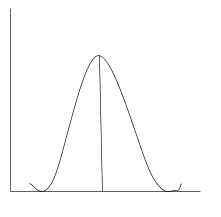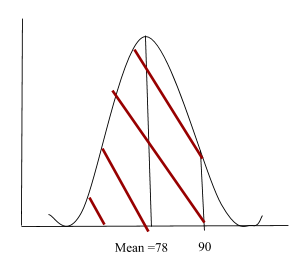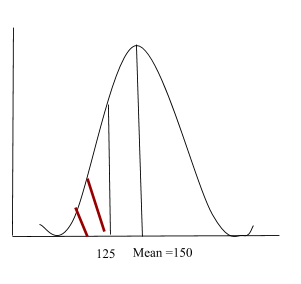# The Normal Distribution

## Summary

• The normal distribution has a bell shaped curve
• It is symmetric about the mean
• Each normal distribution is affected by the either the mean($\mu$) or standard deviation($\sigma$)
• The total area under the normal curve is equal to 1
• To find the normal distribution we use the following formula:$z\quad =\quad \frac { x\quad -\quad \mu }{ \sigma }$

The normal distribution is a theoretical distribution of values. You will already be familiar with its bell shaped curve(shown below).

The normal distribution has many characteristics such as its single peak, most of the data value occurs near the mean, thus a single peak is produced in the middle. Secondly, it is symmetric about the mean. That is, the distributions of values to the right and left of the mean are mirror images, which shows that the distribution, lastly, tapering. Meaning, the further you get from the mean the fewer data is represented thus, it can be said that the distribution tapers out in both direction.

The graph below shows the probability density function of normal distribution.Normal distribution is affected by various factors. That is, whenever there are several different factors, affecting the outcome. We tend to produce a graph like normal distribution.

Moreover, each normal distribution is affected by the either the mean($\mu$) or standard deviation($\sigma$).$N(\mu ,\quad { \sigma }^{ 2 })$.

In normal distribution, mean is also considered the median as it tells us the midpoint of the graph. As it is shown in the graph below, the line of symmetry.In order to find the normal distribution we use the following formula:$z\quad =\quad \frac { x\quad -\quad \mu }{ \sigma }$

#### Example #1

The average on a statistics test was 78 with a standard deviation of 8. If the test results are normally distributed, find the probability that a student receives a test score less than 90.

Step 1

We know that average is also known as mean. So our mean is 78 and are standard deviation is 8. We also know that 90 is more than the mean, so it will be drawn to the right of the mean. We will now draw our normal distribution curve. And find the value of the shaded region.Step 2

We will now, put both the values in the formula. To find the normal distribution of P(X < 90)$z\quad =\quad \frac { x\quad -\quad \mu }{ \sigma } \quad =\quad \frac { 90\quad -\quad 78 }{ 8 } \quad =\quad 1.5$

Step 3

We will check the value P(X < 90) = P(X < 1.5) from our z score table, under 1.5 and get the answer 0.9332.

We can thus conclude our answer by saying, the probability that a student receives a test score less than 90 is 0.9332.

#### Example #2

The average height of 120 students of class 8 in a school is 150cm and the standard deviation is 10cm. Assuming that the height is normally distributed, determine how many students are more than 170cm and less than 12 cm.

Step 1

In this problem the data given to us is as follows:

mean = μ = 150

standard deviation = σ = 10

We will first draw our normal distribution graph. Just like we did in the previous example.Since, we are looking for a value greater the 170 cm thus our graph will look like above, and we will subtract 1 from the final answer to get the value of greater than 170 cm.

Step 2

We will plug in the values in the formula.

The z score for 170cm would be:$z\quad =\quad \frac { x\quad -\quad \mu }{ \sigma } \quad =\quad \frac { 170\quad -\quad 150 }{ 10 } \quad =\quad 2$

Step 3

From the z score table we find that:

P(z ≥ 2)

= 1−0.977251

= 0.02275

Thus the number of students having height more than 170cm can be expected to be:

0.02275×120 = 2.73

≈ 3 students

b)The z score for 125cm is:$z\quad =\quad \frac { x\quad -\quad \mu }{ \sigma } \quad =\quad \frac { 125\quad -\quad 150 }{ 10 } \quad =\quad -2.5$

From the normal distribution z score table we find that the P value for z = −2.5 is:

P(z ≤ −2.5) = 0.00621

Thus the number of students having height less than 125 cm would be:

0.00621 × 120 = 0.7452

≈1

So we cannot expect more than 1 student to have a height less than 125 cm.